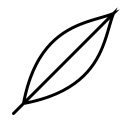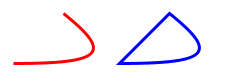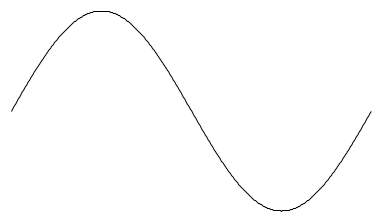# Paths

## Concept

Paths are a way to construct complex shapes, implemented by the Path algebra and the OpenPath ClosedPath types. A path specifies how to control a virtual pen to draw a line. There are three different commands that a path can contain:

• straight lines;
• bezier curves; and
• straight line movement that doesn't draw to the screen.

Here's an example of a path. Notice that it has examples of all of the different components above.

``````import doodle.core._
import doodle.java2d._
import doodle.syntax.all._

val feather =
ClosedPath.empty
.lineTo(100, 100)
.curveTo(90, 75, 90, 25, 10, 10)
.moveTo(100, 100)
.curveTo(75, 90, 25, 90, 10, 10)
.path``````

Drawing this path creates the output below.You probably noticed this is a `ClosedPath`, which suggests there is also an `OpenPath`. This is correct. The difference is how the path ends. If a `ClosedPath` doesn't end at the point it started (which is the origin), it will have a straight line inserted joining the end to the start. An `OpenPath` will not.

Here's an example showing the difference. We create a curve, drawn as an open and a closed path. Notice how the open path is just a curve, while the closed path has an extra line added joining the start and end points.

``````val open =
OpenPath.empty.curveTo(90, 0, 100, 10, 50, 50).path.strokeColor(Color.red)

val closed =
ClosedPath.empty.curveTo(90, 0, 100, 10, 50, 50).path.strokeColor(Color.blue)

val curves = open.beside(closed)``````## Implementation

ClosedPath and OpenPath are both part of `doodle.core`, and not tied to either algebras or `Image`.

You can create a path by calling the `empty` method on either `ClosedPath` or `OpenPath`, and then calling methods on the resulting object. This is the approach used above. You can also creates instances of PathElement and create a path from a `List[PathElement]`.

To convert a path to a `Picture` you can use the `path` syntax method, which is demonstrated in the examples above, or use the `path` method on the `Picture` object.

To create an `Image` from a path, use the `path` method on `Image`. Here's an example.

``````import doodle.image._

val openPath = OpenPath.empty.lineTo(100, 100)

val closedPath = ClosedPath.empty.lineTo(100, 100)

val path1 = Image.path(openPath)
val path2 = Image.path(closedPath)``````

## Utilities

There are several utilities to create common shapes. These are available as both `Picture` and `Image` constructors.

• `equilateralTriangle(width)` creates an equilateral triangle with the given side length.
• `regularPolygon(sides, radius)` creates a regular polygon with the given number of sides and radius.
• `star(points, outerRadius, innerRadius)` creates a star with the given number of points. The points extend as far as `outerRadius` and go in to `innerRadius`.
• `rightArrow(width, height)` creates an arrow points to the right with the given width and height.
• `roundedRectangle(width, height, radius)` creates a rectangle of the given width and height, with rounded corners with size given by `radius`.

These, and other methods, also exist on `OpenPath` and `ClosedPath`. You can also create these paths as a `List[PathElement]` by calling the methods on PathElement.

There is also `interpolatingSpline`, which creates a curve that intersects a given sequence of points. Here's an example.

``````val points =
for (x <- 0.to(360)) yield Point(x, x.degrees.sin * 100)

val curve = Picture.interpolatingSpline(points.toList)``````Layout→